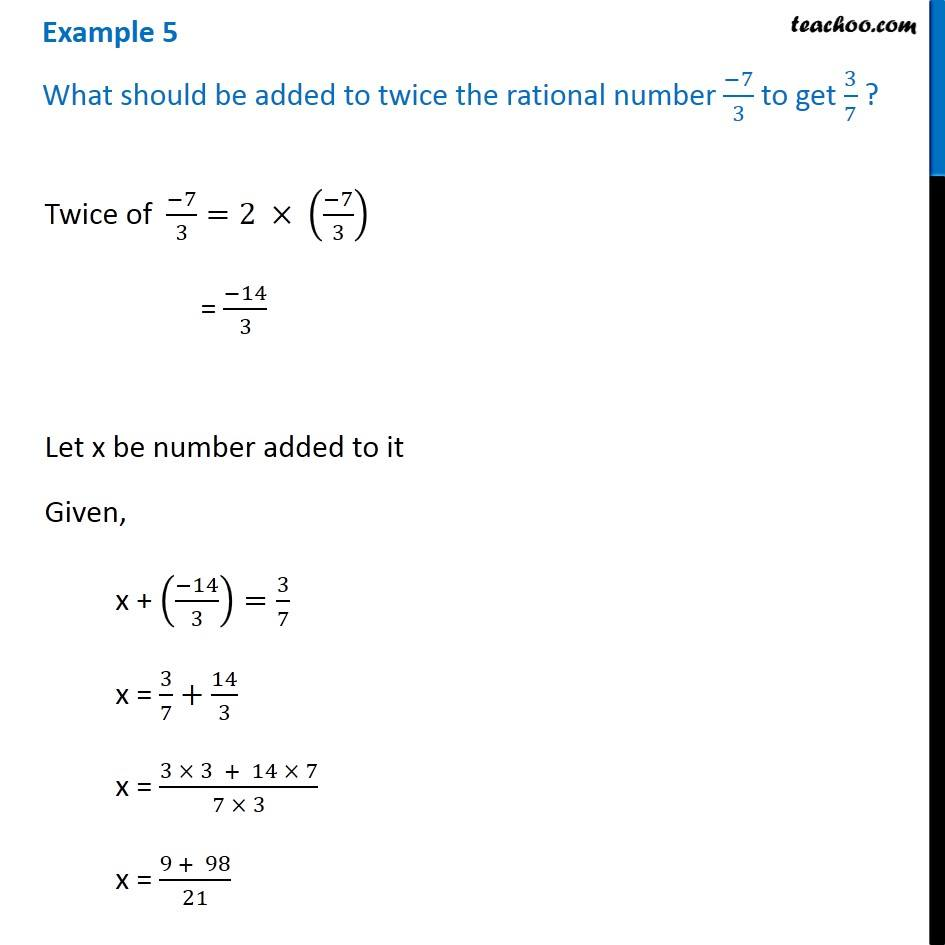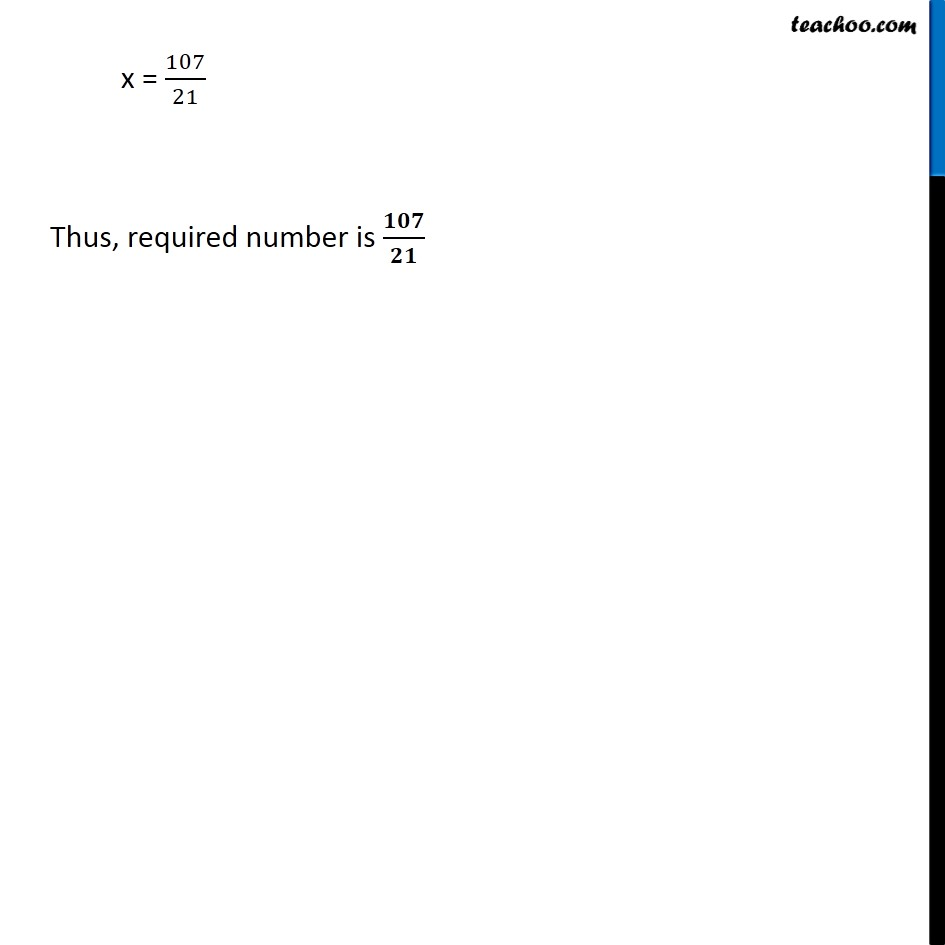1. Chapter 2 Class 8 Linear Equations in One Variable
2. Concept wise
3. Forming Linear Equations - Adding subtracting numbers

Transcript

Example 5 What should be added to twice the rational number ( 7)/3 to get 3/7 ?Twice of ( 7)/3=2 (( 7)/3) = ( 14)/3 Let x be number added to it Given, x + (( 14)/3)=3/7 x = 3/7+14/3 x = (3 3 + 14 7)/(7 3) x = (9 + 98)/21 x = 107/21 Thus, required number is /

Forming Linear Equations - Adding subtracting numbers